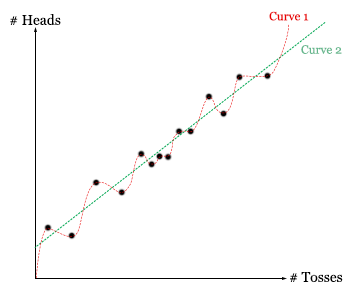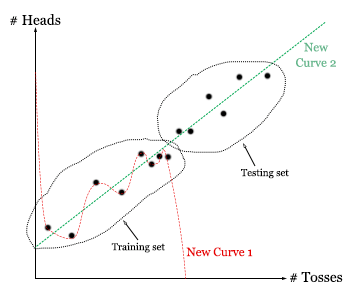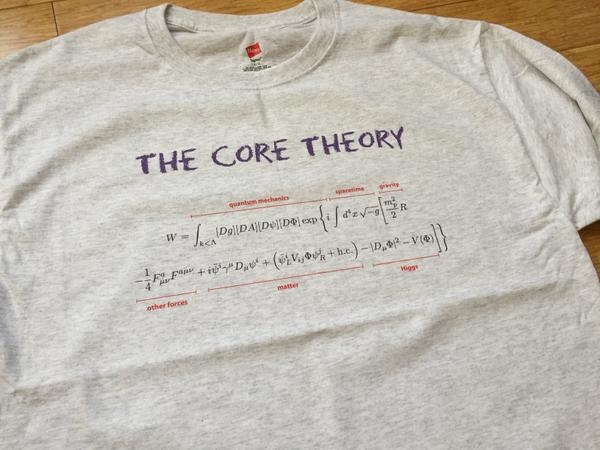# Inference as a balance of accommodation, prediction, and simplicity

(This post is a soft intro to some of the many interesting aspects of model selection. I will inevitably skim over many nuances and leave out important details, but hopefully the final product is worth reading as a dive into the topic. A lot of the general framing I present here is picked up from Malcolm Forster’s writings.)

What is the optimal algorithm for discovering the truth? There are many different candidates out there, and it’s not totally clear how to adjudicate between them. One issue is that it is not obvious exactly how to measure correspondence to truth. There are several different criterion that we can use, and in this post, I want to talk about three big ones: accommodation, prediction, and simplicity.
The basic idea of accommodation is that we want our theories to do a good job at explaining the data that we have observed. Prediction is about doing well at predicting future data. Simplicity is, well, just exactly what it sounds like. Its value has been recognized in the form of Occam’s razor, or the law of parsimony, although it is famously difficult to formalize.
Let’s say that we want to model the relationship between the number of times we toss a fair coin and the number of times that it lands H. We might get a data set that looks something like this:Now, our goal is to fit a curve to this data. How best to do this?

Consider the following two potential curves:Curve 1 is generated by Procedure 1: Find the lowest-order polynomial that perfectly matches the data.

Curve 2 is generated by Procedure 2: Find the straight line that best fits the data.

If we only cared about accommodation, then we’ll prefer Curve 1 over Curve 2. After all, Curve 1 matches our data perfectly! Curve 2, on the other hand, is always close but never exactly right.

On the other hand, regardless of how well Curve 1 fits the data, it entirely misses the underlying pattern in the data captured by Curve 2! This demonstrates one of the failure modes of a single-minded focus on accommodation: the problem of overfitting.

We might want to solve in this problem by noting that while Curve 1 matches the data better, it does so in virtue of its enormous complexity. Curve 2, on the other hand, matches the data pretty well, but does so simply. A combined focus on accommodation + simplicity might, therefore, favor Curve 2. Of course, this requires us to precisely specify what we mean by ‘simplicity’, which has been the subject of a lot of debate. For instance, some have argued that an individual curve cannot be said to be more or less simple than a different curve, as just rephrasing the data in a new coordinate system can flip the apparent simplicity relationship. This is a general version of the grue-bleen problem, which is a fantastic problem that deserves talking about in a separate post.

Another way to solve this problem is by optimizing for accommodation + prediction. The over-fitted curve is likely to be very off if you ask for predictions about future data, while the straight line is likely going to do better. This makes sense – a straight line makes better forecasts about future data because it has gotten to the true nature of the underlying relationship.

What if we want to ensure that our model does a good job at predicting future data, but are unable to gather future data? For example, suppose that we lost the coin that we were using to generate the data, but still want to know what model would have done best at predicting future flips? Cross-validation is a wonderful technique that can be used to deal with exactly this problem.

How does it work? The idea is that we randomly split up the data we have into two sets, the training set and the testing set. Then we train our models on the training set (see which curve each model ends up choosing as its best fit, given the training data), and test it on the testing set. For instance, if our training set is just the data from the early coin flips, we find the following:Cross validation

We can see that while the new Curve 2 does roughly as well as it did before, the new Curve 1 will do horribly on the testing set. We now do this for many different ways of splitting up our data set, and in the end accumulate a cross-validation “score”. This score represents the average success of the model at predicting points that it was not trained on.

We expect that in general, models that overfit will tend to do horribly badly when asked to predict the testing data, while models that actually get at the true relationship will tend to do much better. This is a beautiful method for avoiding overfitting by getting at the deep underlying relationships, and optimizing for the value of predictive accuracy.

It seems like predictive accuracy and simplicity often go hand-in-hand. In our coin example, the simpler model (the straight line) was also the more predictively accurate one. And models that overfit tend to be both bad at making accurate predictions and enormously complicated. What is the explanation for this relationship?

One classic explanation says that simpler models tend to be more predictive because the universe just actually is relatively simple. For whatever reason, the actual relationships between different variables in the universe happens to be best modeled by simple equations, not complicated ones. Why? One reason that you could point to is the underlying simplicity of the laws of nature.

The Standard Model of particle physics, which gives rise to basically all of the complex behavior we see in the world, can be expressed in an equation that can be written on a t-shirt. In general, physicists have found that reality seems to obey very mathematically simple laws at its most fundamental level.I think that this is somewhat of a non-explanation. It predicts simplicity in the results of particle physics experiments, but does not at all predict simple results for higher-level phenomenon. In general, very complex phenomena can arise from very simple laws, and we get no guarantee that the world will obey simple laws when we’re talking about patterns involving 1020 particles.

An explanation that I haven’t heard before references possible selection biases. The basic idea is that most variables out there that we could analyze are likely not connected by any simple relationships. Think of any random two variables, like the number of seals mating at any moment and the distance between Obama and Trump at that moment. Are these likely to be related by a simple equation? Of course!

(Kidding. Of course not.)

The only times when we do end up searching for patterns in variables is when we have already noticed that some pattern does plausibly seem to exist. And since we’re more likely to notice simpler patterns, we should expect a selection bias among those patterns we’re looking at. In other words, given that we’re looking for a pattern between two variables, it is fairly likely that there is a pattern that is simple enough for us to notice in the first place.

Regardless, it looks like an important general feature of inference systems to provide a good balance between accommodation and either prediction or simplicity. So what do actual systems of inference do?

I’ve already talked about cross validation as a tool for inference. It optimizes for accommodation (in the training set) + prediction (in the testing set), but not explicitly for simplicity.

Updating of beliefs via Bayes’ rule is a purely accommodation procedure. When you take your prior credence P(T) and update it with evidence E, you are ultimately just doing your best to accommodate the new information.

Bayes’ Rule: P(T | E) = P(T) ∙ P(E | T) / P(T)

The theory that receives the greatest credence bump is going to be the theory that maximizes P(E | T), or the likelihood of the evidence given the theory. This is all about accommodation, and entirely unrelated to the other virtues. Technically, the method of choosing the theory that maximizes the likelihood of your data is known as Maximum Likelihood Estimation (MLE).

On the other hand, the priors that you start with might be set in such a way as to favor simpler theories. Most frameworks for setting priors do this either explicitly or implicitly (principle of indifference, maximum entropy, minimum description length, Solomonoff induction).

Leaving Bayes, we can look to information theory as the foundation for another set of epistemological frameworks. These are focused mostly on minimizing the information gain from new evidence, which is equivalent to maximizing the relative entropy of your new distribution and your old distribution.

Two approximations of this procedure are the Akaike Information Criterion (AIC) and the Bayesian Information Criterion (BIC), each focusing on subtly different goals. Both of these explicitly take into account simplicity in their form, and are designed to optimize for both accommodation and prediction.

Here’s a table of these different procedures, as well as others I haven’t mentioned yet, and what they optimize for:

 Optimizes for… Accommodation? Prediction? Simplicity? Maximum Likelihood Estimation ✔ Minimize Sum of Squares ✔ Bayesian Updating ✔ Principle of Indifference ✔ Maximum Entropy Priors ✔ Minimum Message Length ✔ Solomonoff Induction ✔ P-Testing ✔ ✔ Minimize Mallow’s Cp ✔ ✔ Maximize Relative Entropy ✔ ✔ Minimize Log Loss ✔ ✔ Cross Validation ✔ ✔ Minimize Akaike Information Criterion (AIC) ✔ ✔ Minimize Bayesian Information Criterion (AIC) ✔ ✔

Some of the procedures I’ve included are closely related to others, and in some cases they are in fact approximations of others (e.g. minimize log loss ≈ maximize relative entropy, minimize AIC ≈ minimize log loss).

We can see in this table that Bayesianism (Bayesian updating + a prior-setting procedure) does not explicitly optimize for predictive value. It optimizes for simplicity through the prior-setting procedure, and in doing so also happens to pick up predictive value by association, but doesn’t get the benefits of procedures like cross-validation.

This is one reason why Bayesianism might be seen as suboptimal – prediction is the great goal of science, and it is entirely missing from the equations of Bayes’ rule.

On the other hand, procedures like cross validation and maximization of relative entropy look like good candidates for optimizing for accommodation and predictive value, and picking up simplicity along the way.